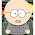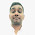## Saturday, October 29, 2011

### I've Got the Power (Law)

You've got the power!

What's the Power Law?
I'm glad you asked, although I don't know a whole bunch about it. If you want a deeper look, check out Transforming Classroom Grading, which goes into the research behind the Power Law of Learning and provides a step-by-step for using it in calculations. But, lemme sum up.

If you were to plot out the scores of a student learning a skill which was brand new to them, which graph do you think would represent their scores over time---the one on the left...or the one on the right?Inquiring optometrists want to know: "Which looks more clear: #1 or #2?"

Both lines have the same start and end point. They describe the same number of assignments. But one is a better representation for what happens during the learning process. Did you guess the one on the right? Yup. You were correct. When new skills are introduced, there is a big gain in learning at the beginning. With repeated opportunities, learning still increases, but at a much slower rate. Devotees of the Power Law believe that the most recent score for a student is the what should be reported as a grade.

Okay. So now what?
Oh, that student scores followed the exact same trajectory every time. But that's not what happens. Learning is impacted by all sorts of factors both inside and outside of the classroom. The graph above shows the average increase. We need to be able to make an individual student's scores apply to that learning curve. This is where the Power Law comes in handy. Here it is:Hello...Newman...

It might have been many moons since high school algebra, but perhaps the Power Law reminds you of the one used for slope: y = mx + b. Makes sense as we are trying to look for a trend in learning. In our Power Law formula, y is the student score that is predicted and x is the original student score. The roles of m and b are to act as constants. They take into account the number of scores for a given standard and the position of a score within that range.

Calculating m and b requires some statistics, because in a way, we're "normalizing" the data set to the curve. If it's been awhile since you had to deal with this sort of thing, remember that the "E" symbol means "sum of" and "N" = "Number" (in this case, referring to the number of assignments for a given standard. Don't get too sweaty looking at these, because our buddy Excel is going to do all of the heavy lifting for you. I just want you to see them before we look at the gradebook.omgwtfbbq

And now, a musical interlude:

Back to our regularly scheduled workbook...

I'm going to build this workbook using the finalized Excel for Beginners workbook. Keep in mind that these formulas will work the Intermediate and Advanced versions, too. Just plop the formulas any old place and they'll do your bidding. Here is the first chunk of student scores:

Now, we need to transform them using the Power Law. I've set up a column for the variables we're going to use: x = (natural log of) Assignment #, y = (natural log of) Student Score, then the various combos we'll need using these. Please note that the y here is not the same as the one in the Power Law itself---it is just a statistical variable assigned to calculate m and b. (Confused yet?)

And here are the formulas generating these values:

I've probably made this look far more complicated than it needs to be, but I also like the idea of breaking down the steps---especially if you're new to Excel or just need the opportunity to follow along with what is happening. The formulas in D26 and D27 use the natural log (LN) to transform the ordinal number of the assessment (x) and the student score on that assessment (y). Cells D28 - D34 are used to process x and y so they are ready to use in our equations for m (D35) and b (D36). The "predicted score" showing in Row 22 shows you the result of the Power Law formula (y = mx^b) for each student score.

Here is the link for the Power Law workbook with formulas. We'll talk about the graphs for this function in the next post, as well as reveal the final version of the workbook. Download this one first so you have something to play with.

Now, come on and sing it with me:

Bonus Round
Want more of The Power by Snap! to listen to while you work on your gradebook? Cue this up.

1.Un millón de gracias!

2.If we look carefully at the graphics you show, there's a serious disconnect. The student scores do not increase over time, but the next example, which shows how the formula works, has student scores that increase. I was really interested to see how you would suggest figuring out which assignments should be used in the trending, but this graphic has "homework," "homework," "quiz," "homework," "lab," "homework," and "test" listed. Are we to believe these all measured the exact same skill in the same way and with the exact same level of difficulty? If not, the trending is deeply unfair. If so, that teacher had to essentially give the same assignment to students 6 times to measure that one skill in order to measure a trend, which seems like a huge waste of both the students' and teacher's time. As our district's proficiency guru pointed out to me, for trending to be useful, you'd have to break down each standard into its component skills, then measure each one in the same way at least four times over the course of the semester. With, say, 40 CCSS in LA, that would mean 160 assignments per student per semester. I have 197 kids, so that would be 31,520 grades per semester, plus redos. Our guru says this would be a better way to do proficiency, but I'd like to carve out some time for teaching from all the assessment I've been doing. Though trending sounds better in theory, besides being more difficult to explain to parents, I think it requires a kind of teaching that's bad for kids and impossible for teachers. Am I missing something?

1.No you are not missing something. The whole need for "power law" calculations is a byproduct of the limited scale (4 points) used for EACH and EVERY assignment. 15 minute quiz -- 4 point score recorded in the grade book. 50 minute test -- 4 point score recorded in the grade book. This is supposed to improve the "reliability" of each assessment; but all it really does is hide all the messy, inaccuracy of this process by pretending there is come true "curve" that is discovered through mathematical wizardry.

Basically, this approach give more weight to assessments that come later in the series. Gee ... kind of like the old classwork (very little weight), quiz (a bit more weight), test (a whole bunch of weight) approach that teachers have been using for years.

3.Does your excel file with the power law factor in an adjustment for time differences between assessments?

4.Hi what is that standard and how do you calculate it? Thanks

5.Are there any Kindergarten 5 year olds Teacher?
What type assessments do you use?

6.Hello, I would be in urgent need (24 hours), for a math related research in this field, to access the procedure which leads to the final formula. I'm in Italy and the book you suggest in this page would take several days to ship. An e-book is not available. Could you please help? In several versions of the formula online I find 4 variables (x,s,y,N). I am under the impression that there is one too many. In the formula you have applied how many variables do you have....3? (X,y,N, and you can calculate m and b). Could you please write the full formula below for me to check my results? Thanks a lot..

7.Hi, Milena, if you look above in the post at the graphics, you can see the whole thing.

y = mx^b

The graphic below shows how you calculate m and b for the formula. This assumes you have a set of scores (N).